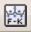# HRFK

## High resolution frequency wavenumber Toolbox (Capon's method) - overview

The high resolution frequency wavenumber (hrfk) algorithm follows the ideas of Capon (1969) . It can be viewed as a generalized beamforming algorithm resulting in an auto-adaptive (optimal) complex spatial weighting scheme. for analysis of narrowband stationary signals, it is one of the most common and preferred frequency wavenumber techniques applied to ambient vibration analysis.

The Capon beamformer relies on a very simple formula:

$BP_{Capon}(\omega ,{\vec {k}})={\frac {1}{{\vec {e}}^{\dagger }(\omega ,{\vec {k}}){\underline {R}}^{-1}(\omega ){\vec {e}}(\omega ,{\vec {k}})}}$where ${\underline {R}}^{-1}(\omega )$is simply the inverse of the cross spectral matrix estimate and ${\vec {e}}$is the so-called steering vector summarizing the shift times for the harmonic plane wave with wavenumber vector ${\vec {k}}$for each station within the array.The $\dagger$symbol presents the conjugate transpose operation. In the same notation, the conventional f-k algorithm would be formulated as:

$BP_{conv.}(\omega ,{\vec {k}})={\vec {e}}^{\dagger }(\omega ,{\vec {k}}){\underline {R}}(\omega ){\vec {e}}(\omega ,{\vec {k}})$For further details, we refer you to the array signal processing page of this wiki. Here we only emphasize, that the inverse of the cross-spectral matrix has to be computed for the high-resolution technique, making the estimate potentially unstable.

The corresponding toolbox for the high-resolution frequency wavenumber technique can be opened using the following plugin icon. The toolbox is nearly equivalent to the conventional frequency wavenumber toolbox and the processing flow is exactly equivalent to the conventional technique.

## Parameter settings

Compared to the conventional f-k toolbox, there is just one single (optional!) parameter that is included in the processing tab of the toolbox. As the calculation for the hrfk algorithm involves the inversion of the cross spectral matrix estimate from the data (see above), it may sometimes be necessary to stabilize the cross spectral matrix before inverting.

The stabilization can be achieved by diagonal loading, sometimes also referred to by damping using a regularization parameter. The damping factor is the new optional parameter in the high-resolution frequency wavenumber toolbox. A good value is usually found by trial and error, but a good starting value is probably 0.001 to 0.01.

Note that the usage of the damping factor is optional and should be omitted when possible. When using the damping factor, you will implicitly smooth out your wavenumber power spectra and eventually not obtain much better results compared to the conventional beamforming approach (considering resolution).

The better and data driven approach for obtaining a stable (non-singular) cross spectral matrix estimate is achieved via temporal or spectral smoothing of the spectral covariance matrix. The temporal smoothing consists of averaging cross spectral estimates from distinct short time windows and is therefore also referred to as block averaging or direct segment method. An equivalent implementation can be achieved by smoothing over neighbouring frequency samples in the Fourier domain after obtaining one spectral estimate on a long time analysis window. The implementation in Geopsy follows the second option.

Capon (1969) states that the number of blocks $N_{blocks}$to be averaged must be equal or higher than the number of stations $M$within the array to guarantee a regular cross spectral matrix estimate from the data. Equivalently, the number of frequency samples $N_{freq}$to be averaged must equal at least the number of stations in the array.

$N_{blocks}\geq M\quad {\textrm {or}}\quad N_{freq}\geq M$It is clear, that for a given window length and a specified bandwidth parameter, the number of Fourier coefficients may be too small to meet the criterion above. If this is the case, the hrfk will refuse to do any computation on the data set. One will notice this behavior when checking the result in the wavenumber window browser. It will show a plain magenta color. If you experience this problem you should either increase the length of your analysis window or increase the bandwidth of processing. Most probably you would like to increase your window length though, as increasing the bandwidth will tend to smooth out results as you are capturing waves traveling indeed with different phase velocities. The bandwidth parameter typically should be not larger than 0.05, the window length can be set to 200 to 500 cycles.

## Contents of output file

The output files for the high resolution f-k approach are equivalent and compatible to the output files produced with the conventional approach. For details check the following page.

## Graphical display of high resolution f-k results using max2curve

The usage of max2curve is equivalent to using this tool with .max files produced with the conventional f-k approach. Check also the following page for details.